# 一族链环的Alexander多项式Alaxander Polynomials a Family of Links

DOI: 10.12677/AAM.2019.811201, PDF, HTML, XML, 下载: 249  浏览: 348

Abstract: Pretzel link is a kind of special and simple link. This paper studies a kind of special directional Pretzel link P（c1，c2，···，cn）, gives P（c1，c2，···，cn） Seifert surface and the property of Seifert matrix, and finds the Alexander polynomial calculation formula of this family of Pretzel link.

1. 引言

2. 预备知识

2.1. 纽结

2.2. 投影图

2.3. 链环

2.4. Seifert曲面

2.5. 交叉数

2.6. Seifert矩阵

${v}_{jk}=lk\left({a}_{j}^{-},{a}_{k}\right)$${a}_{j}^{-},{a}_{k}$ 的交错数，记 $V=\left({v}_{jk}\right)$ 为K的Seifert矩阵。

2.7. 定理1

$V=\left({v}_{jk}\right)$ 为K的Seifert矩阵，那么 $A\left(t\right)={V}^{\text{T}}-tV$ 就是K的Alexander矩阵，Alexander矩阵与Alexander多项式的关系是： $\Delta \left(t\right)=\mathrm{det}A\left(t\right)$ 。

3. 定理证明

$\begin{array}{c}\mathrm{det}A\left(t\right)\\ ={\left(1-t\right)}^{n-1}|\begin{array}{cccccc}{K}_{1}+{K}_{2}& -{K}_{1}& {K}_{1}& \cdots & \cdots & {\left(-1\right)}^{n}{K}_{1}\\ {K}_{2}& K{}_{3}& & & & \\ & {K}_{3}& {K}_{4}& & & \\ & & & & & ⋮\\ & & & \ddots & & \\ & & & {K}_{n-2}& {K}_{n-1}& \\ & & & & {K}_{n-1}& {K}_{n}\end{array}|\\ ={\left(1-t\right)}^{n-1}\underset{i=1}{\overset{t}{\sum }}{K}_{1}{K}_{2}\cdots {\stackrel{¯}{K}}_{i}\cdots {K}_{n}\end{array}$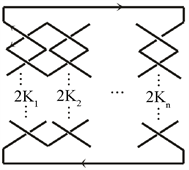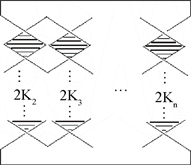Figure 2. Seifert surface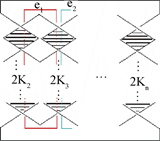Figure 3. Generator of a seifert surfaceFigure 4. The winding method of ${e}_{1}{e}_{1}^{+}$

${e}_{1}{e}_{2}^{+}$ 的缠绕方式如图5所示所以交叉数为 ${K}_{2}$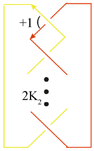Figure 5. The winding method of ${e}_{1}{e}_{2}^{+}$

${e}_{2}{e}_{1}^{+}$ 的缠绕方式如图6所示，所以交叉数为 ${K}_{2}$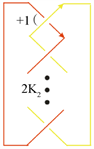Figure 6. The winding method of ${e}_{2}{e}_{1}^{+}$

$V=\left[\begin{array}{cccccc}{K}_{1}+{K}_{2}& {K}_{2}& & & & \\ {K}_{2}& {K}_{2}+{K}_{3}& {K}_{3}& & & \\ & & & & & \\ & & \ddots & & & \\ & & & & & \\ & & & {K}_{n-2}& {K}_{n-2}+{K}_{n-1}& {K}_{n-1}\\ & & & & {K}_{n-1}& {K}_{n-1}+{K}_{n}\end{array}\right]$

$\begin{array}{c}A\left(t\right)={V}^{\text{T}}-tV\\ ={\left(1-t\right)}^{n-1}\left[\begin{array}{cccccc}{K}_{1}+{K}_{2}& {K}_{2}& & & & \\ {K}_{2}& {K}_{2}+{K}_{3}& {K}_{3}& & & \\ & & & & & \\ & & & \ddots & & \\ & & & & & \\ & & & {K}_{n-2}& {K}_{n-2}+{K}_{n-1}& {K}_{n-1}\\ & & & & {K}_{n-1}& {K}_{n-1}+{K}_{n}\end{array}\right]\end{array}$

$\mathrm{det}A\left(t\right)={\left(1-t\right)}^{n-1}|\begin{array}{cccccc}{K}_{1}+{K}_{2}& {K}_{2}& & & & \\ {K}_{2}& {K}_{2}+K{}_{3}& {K}_{3}& & & \\ & & & & & \\ & & \ddots & & & \\ & & & & & \\ & & & {K}_{n-2}& {K}_{n-2}+{K}_{n-1}& {K}_{n-1}\\ & & & & {K}_{n-1}& {K}_{n-1}+{K}_{n}\end{array}|$

$\mathrm{det}A\left(t\right)$ 的第一列 $×\left(-1\right)$ 加到第二列，再将变形后的第二列 $×\left(-1\right)$ 加到第三列，按照此方法一直到 $n-1$ 列，即得到：

$\mathrm{det}A\left(t\right)={\left(1-t\right)}^{n-1}|\begin{array}{cccccc}{K}_{1}+{K}_{2}& -{K}_{1}& {K}_{1}& \cdots & \cdots & {\left(-1\right)}^{n}{K}_{1}\\ {K}_{2}& K{}_{3}& & & & \\ & {K}_{3}& {K}_{4}& & & \\ & & {}_{}& & & ⋮\\ & & & \ddots & & \\ & & & {K}_{n-2}& {K}_{n-1}& \\ & & & & {K}_{n-1}& {K}_{n}\end{array}|$

$n=3$$\mathrm{det}A\left(t\right)={\left(1-t\right)}^{2}|\begin{array}{cc}{K}_{1}+{K}_{2}& {K}_{2}\\ {K}_{2}& {K}_{2}+{K}_{3}\end{array}|={K}_{2}{K}_{3}+{K}_{1}{K}_{3}+{K}_{1}{K}_{2}$ 显然成立。

$n=N$ 则有

$\mathrm{det}A\left(t\right)={\left(1-t\right)}^{N-1}|\begin{array}{cccccc}{K}_{1}+{K}_{2}& -{K}_{1}& {K}_{1}& \cdots & \cdots & {\left(-1\right)}^{N}{K}_{1}\\ {K}_{2}& K{}_{3}& & & & \\ & {K}_{3}& {K}_{4}& & & \\ & & & & & ⋮\\ & & & \ddots & & \\ & & & {K}_{N-2}& {K}_{N-1}& \\ & & & & {K}_{N-1}& {K}_{N}\end{array}|=\underset{i=1}{\overset{n}{\sum }}{K}_{1}{K}_{2}\cdots {\stackrel{¯}{K}}_{i}\cdots {K}_{N}$

$n=N+1$ 时按第n行展开

$\begin{array}{c}\mathrm{det}A\left(t\right)={\left(1-t\right)}^{N}|\begin{array}{cccccc}{K}_{1}+{K}_{2}& -{K}_{1}& {K}_{1}& \cdots & \cdots & {\left(-1\right)}^{N+1}{K}_{1}\\ {K}_{2}& K{}_{3}& & & & \\ & {K}_{3}& {K}_{4}& & & \\ & & & & & ⋮\\ & & & \ddots & & \\ & & & {K}_{N-1}& {K}_{N}& \\ & & & & {K}_{N}& {K}_{N+1}\end{array}|\\ ={\left(1-t\right)}^{N}{K}_{N+1}\left(\underset{i=1}{\overset{N}{\sum }}{K}_{1}{K}_{2}\cdots {\stackrel{¯}{K}}_{i}\cdots {K}_{N}\right)\\ +{\left(1-t\right)}^{N}{\left(-1\right)}^{2N-1}{K}_{N}|\begin{array}{ccccc}{K}_{1}+{K}_{2}& -{K}_{1}& & & {\left(-1\right)}^{N+1}{K}_{1}\\ {K}_{2}& {K}_{3}& & & \\ & & & & ⋮\\ & & \ddots & & ⋮\\ & & {K}_{N-2}& {K}_{N-1}& \\ & & & {K}_{N-1}& 0\end{array}|\\ ={\left(1-t\right)}^{N}{K}_{N+1}\left(\underset{i=1}{\overset{N}{\sum }}{K}_{1}{K}_{2}\cdots {\stackrel{¯}{K}}_{i}\cdots {K}_{N}\right)+{\left(1-t\right)}^{N}{K}_{1}{K}_{2}\cdots {K}_{N}\\ ={\left(1-t\right)}^{N}\underset{i=1}{\overset{N+1}{\sum }}{K}_{1}{K}_{2}\cdots {\stackrel{¯}{K}}_{i}\cdots {K}_{N+1}\end{array}$

  Adams, C.C. (2004) The Knot Book: An Elementary Introduction to the Mathematical Theory of Knots. The American Mathematical Society, Providence.  Collins, J. (2016) An algorithm for Computing the Seifert Matrix of a Link from a Braid Representation. Ensaios Matemáticos, 30, 246-262.  Kauffman, L.H. and Lopes, P. (2017) Infinitely Many Prime Knots with the Same Alexander Invariants. Journal of Knot Theory and Its Ramifications, 26, Article ID: 1743009. https://doi.org/10.1142/S021821651743009X  Landvoy, R.A. (1998) The Jones Polynomial of Pretzel Knots and Links. Topology and Its Applications, 83, 135-147. https://doi.org/10.1016/S0166-8641(97)00100-4